# Some Refinements to the Gimbal Sim

#### Mick West

Staff member
There are two issues with the Gimbal simulation, which I think I've now resolved.

1 - The difference between the cloud horizon and the artificial horizon over the first 22 seconds
2 - The possible slight apparent continuous clockwise rotation of the object over the first 22 seconds

Both these issues only affect the first 22 seconds and not a lot. They don't make any difference to the four observable that demonstrate we are looking at a rotating glare, except in the sense that it's now both more accurate in terms of the physics and in the end results.

The original Gimbal sim: https://www.metabunk.org/gimbal1/
And the latest one https://www.metabunk.org/gimbal/

The main differences are illustrated by comparing these graphs (old on the left, new on the right)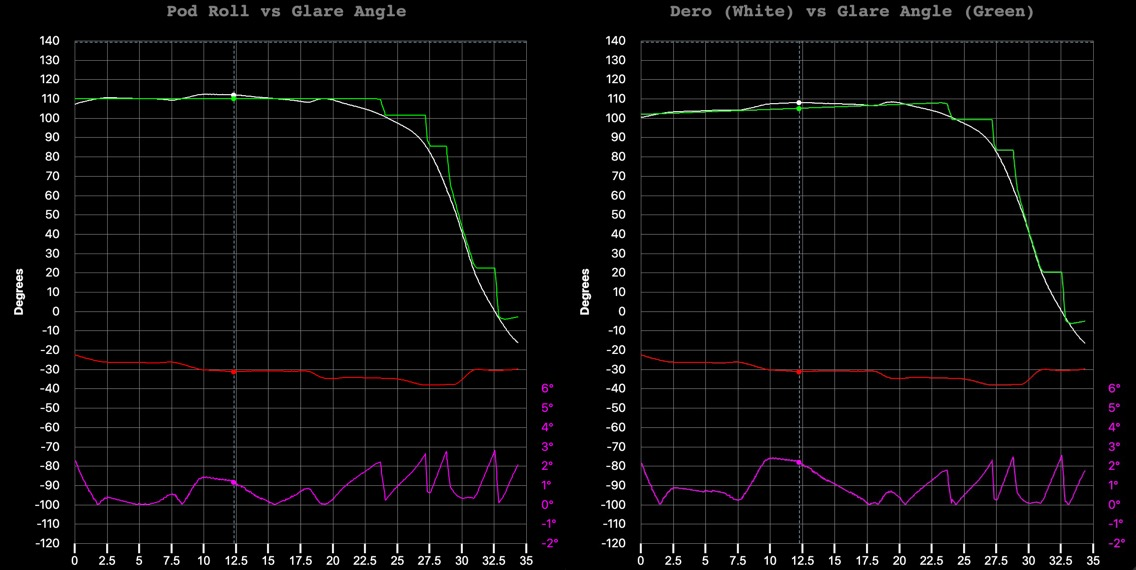And looking at the simulated view, first frame: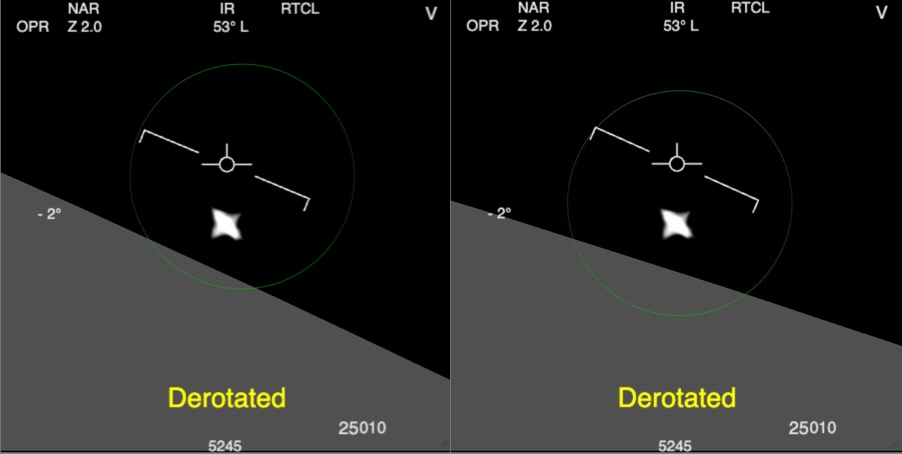The first issue is discussed here:
The short version is that by measuring the direction the clouds moved in (parallel to the horizon), it was clear that this was at a shallower angle than the artificial horizon, most so when looking left.

The reason for this is that the horizon angle in the ATFLIR image should match what the pilot sees when they look in the same direction. I was previously assuming the horizon angle would be the same as the jet's bank angle (i.e. the same as the artificial horizon). But that's only true when looking forward. When looking more to the side then the bank angle contribution is diminished, and you get some more of the pitch angle. The precise math for calculating the desired horizon angle is found here: https://www.metabunk.org/threads/gi...using-clouds-as-the-horizon.12552/post-276183 and explained:
I'm assuming that it's intuitive for pilots to look left/right, up/down, but not to tilt their head, so I try to recreate what the horizon would look like if you had a camera strapped to the jet that can only rotate left/right in the wing plane, or up/down perpendicular to that. First I find how much I need to rotate the camera along those lines to look directly at the object, then I compute the angle between a vector pointing right in the camera plane, and the real horizon given by a vector that is tangential to the global 'az' viewing angle.
(Thanks to @logicbear)

This was easy to implement in Sitrec (not uploaded yet), but the Gimbal Simulator was more complicated. The problem being that the horizon angle (previously the bank angle) is used to calculate the derotation amount, which is equal to the glare angle. This relationship was used in reverse so that the glare angle could drive the pod's rotation (one line of code), and hence necessary derotation, and then it was shown this created a stepped curve (the green curve in the charts above) that closely followed the ideal derotation (the white curve). You see in the chart, it's labeled "pod roll vs. glare angle" since pod roll = deroation.

But with the more accurate and complicated calculation of the desired horizon, there was now the problem of how you go from glare angle to pod roll. Glare angle is still equal to derotation, so you can phrase it as how to find the pod roll for a given derotation.

Essentially what I do is first calculate the pod pitch and roll for the ideal track (the white curve) and then keep everything else constant and do a binary search, varying pod roll, until I get the desired derotation. This new pod roll then physically drives the pod head. In the sim the green dot indicates where the pod head is physically looking. The white dot indicates the actual target, which needs to stay withing 5° of the green dot so that the additional mirrors can keep it centered.

Doing this adjustment gives this graph. The magenta is a magnified measure of the angle between the green and white dots (not the graphed lines, which are dero). It stays under 5°, but it has got worse.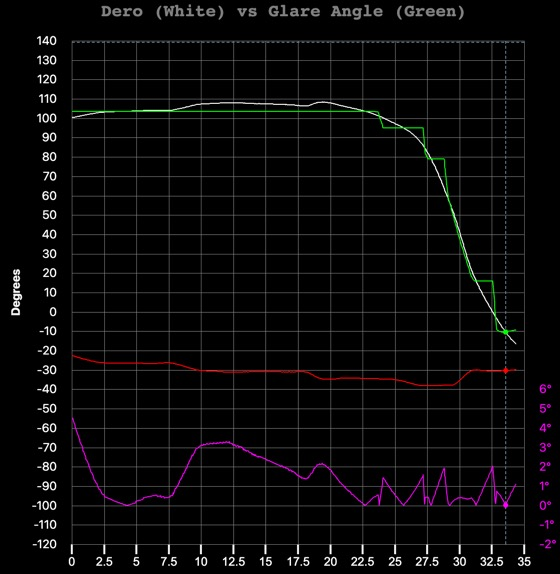This is where the second issue comes in. Many people have argued that the gimbal shape has a slight clockwise rotation over the first 22 seconds. This is hard to measure due to the switching from WHT to BLK, but a good case has been made that it's there. I've not worried too much about it as it does not make a huge difference, but on reviewing it I think it's quite plausible. Incorporating a slight clockwise rotation ends up removing the increased error. The actual amount is not that important, anything from 3° to 16° keeps the error under 4°, but I picked 6°. You can play with this value at "Glare Initial Rotation" under Tweaks.

This was all rather complicated. It's probable that there's an analytical solution to finding roll from dero. But the numerical solution (binary search) is robust, being accurate essentially by definition.
Here's the code for that bit, full new code attached.

JavaScript:
``````// given a jet pitch and roll, and the el and az (the ideal, i.e. the white dod)
// find the pod pitch and roll
// THEN modify roll until the dero for that pitch and roll matches the needed dero for the ideal
// this new roll will give us the green dot (i.e. where the pod head is physically pointing)
function getPodRollFromGlareAngleFrame(frame) {

//   return getGlareAngleFromFrame(frame);

// This is what we want the horizon to be
const humanHorizon = get_real_horizon_angle_for_frame(frame)

// actual Jet orientation
var jetPitch = jetPitchFromFrame(frame) // this will get scaled pitch
var jetRoll = jetRollFromFrame(frame)

// ideal az and el (white dot)
var az = Frame2Az(frame)
var el = Frame2El(frame);

// start pod Pitch and Roll for ideal az and el
var podPitch, totalRoll;
[podPitch, totalRoll] = EAJP2PR(el, az, jetPitch);

var podRoll = totalRoll - jetRoll

// what we want the dero to be
const targetDero = getGlareAngleFromFrame(frame)

// and hence what we want the pod horizon angle to be
const horizonTarget = targetDero + humanHorizon

// binary search here modifying podRoll until the dero calculates from jetRoll, jetPitch, podRoll and podPitch
// is close to targetDero

var rollA = podRoll - 90;
var rollB = podRoll + 90;
var horizonA = getPodHorizonFromJetAndPod(jetRoll, jetPitch, rollA, podPitch)
var horizonB = getPodHorizonFromJetAndPod(jetRoll, jetPitch, rollB, podPitch)
var maxIterations = 1000
while (rollB - rollA > 0.01 && maxIterations-- > 0) {
var rollMid = (rollA+rollB)/2;
var horizonMid = getPodHorizonFromJetAndPod(jetRoll, jetPitch, rollMid, podPitch)
// is the horiozn from A to B increasing or decreasing, that will affect which way we compare
if (horizonB > horizonA) {
// horizon is increasing from A to B
if (horizonTarget < horizonMid) {
// target is in the lower part, so Mid is the new B
rollB = rollMid; horizonB = horizonMid;
} else {
// target is in the upper part, so Mid is the new A
rollA = rollMid; horizonA = horizonMid;
}
} else {
// horizon is decreasing from A to B
if (horizonTarget < horizonMid) {
if (horizonTarget < horizonMid) {
// target is in the smaller upper part, so Mid is the new A
rollA = rollMid;
horizonA = horizonMid;
} else {
// target is in the larger lower part, so Mid is the new B
rollB = rollMid;
horizonB = horizonMid;
}
}
}
}

return rollA;

}``````

And just to reiterate, this does not really change any of the observables. The initial slight continuous rotation does not change the fact when the jet banks, the horizon rotates, but the object does not. And the fourth observable, the derotation matching the glare angle, is still just as accurate as before. The main change is that the simulator now looks even more like the real video in terms of the horizon angle and the slight initial rotation.

#### Attachments

• index.html.zip
38.5 KB · Views: 79
The magnitude and nature of the initial rotation is uncertain, see: https://www.metabunk.org/threads/calculating-and-visualizing-gimbal-angles.12237/post-274060

But it still works regardless of if you do this or not.

@logicbear, did you ever try to extract the gimbal angle from the original file?

I'm just using a linear rotation now. It would be interesting to see if something derived from the video might give different results.

I've incorporated these changes into Sitrec.

@logicbear, did you ever try to extract the gimbal angle from the original file?

I'm just using a linear rotation now. It would be interesting to see if something derived from the video might give different results.
I did switch to the originals but the results didn't change much. With my histogram matching it doesn't seem like there's an obvious discontinuity around the switch from BHT to WHT, but maybe there could be a bit more significant drift that is not entirely detected by the algorithm due to the glare gradually changing shape. Could there also be a more gradual change in the exposure/gain/contrast settings over this period ? Maybe I could try to do some histogram matchings over longer periods, not just at the WHT/BHT boundary.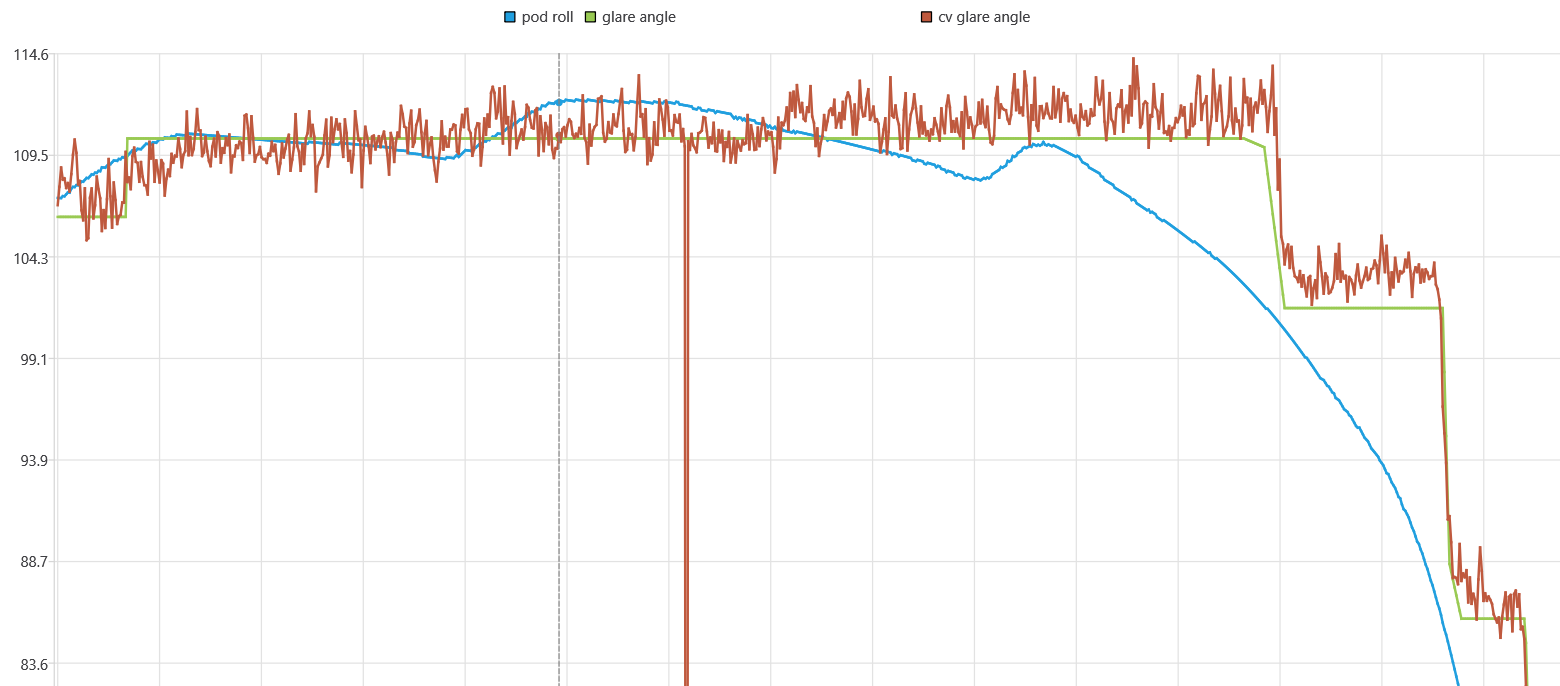The math wasn't too complicated for that. The key was your insight that the horizon should look more intuitive when looking to the side.
find the pod roll for a given derotation ... binary search, varying pod roll, until I get the desired derotation
But doesn't the pod only need to rotate just enough to be able to track the target, and then the desired horizon angle can be set by the derotation device without additional pod roll, so the pod roll shouldn't depend on the desired horizon angle ? Is it possible that the green line with keyframing (with that step I added during the first seconds perhaps) that we had previously was already close to the correct graph for the real pod rotation with steps ? Any additional derotation (without pod roll) due to the desired horizon angle should not affect the error angle graph (magenta) ? Also, shouldn't that error stay within 2.5 degrees so that all of the peaks where the roll motor engages can happen at the same error angle ?

But doesn't the pod only need to rotate just enough to be able to track the target, and then the desired horizon angle can be set by the derotation device without additional pod roll, so the pod roll shouldn't depend on the desired horizon angle ? Is it possible that the green line with keyframing (with that step I added during the first seconds perhaps) that we had previously was already close to the correct graph for the real pod rotation with steps ? Any additional derotation (without pod roll) due to the desired horizon angle should not affect the error angle graph (magenta) ?
Yeah, I think I focussed so much on the math to match to find the pod roll, I forgot the pod should not be rotating. So I think what I'm calculating is essentially actually just the error in the glare angle measurement.

Plotting green = glare angle, white = ideal dero, and yellow = resultant pod roll, with an initial glare rise of 7.2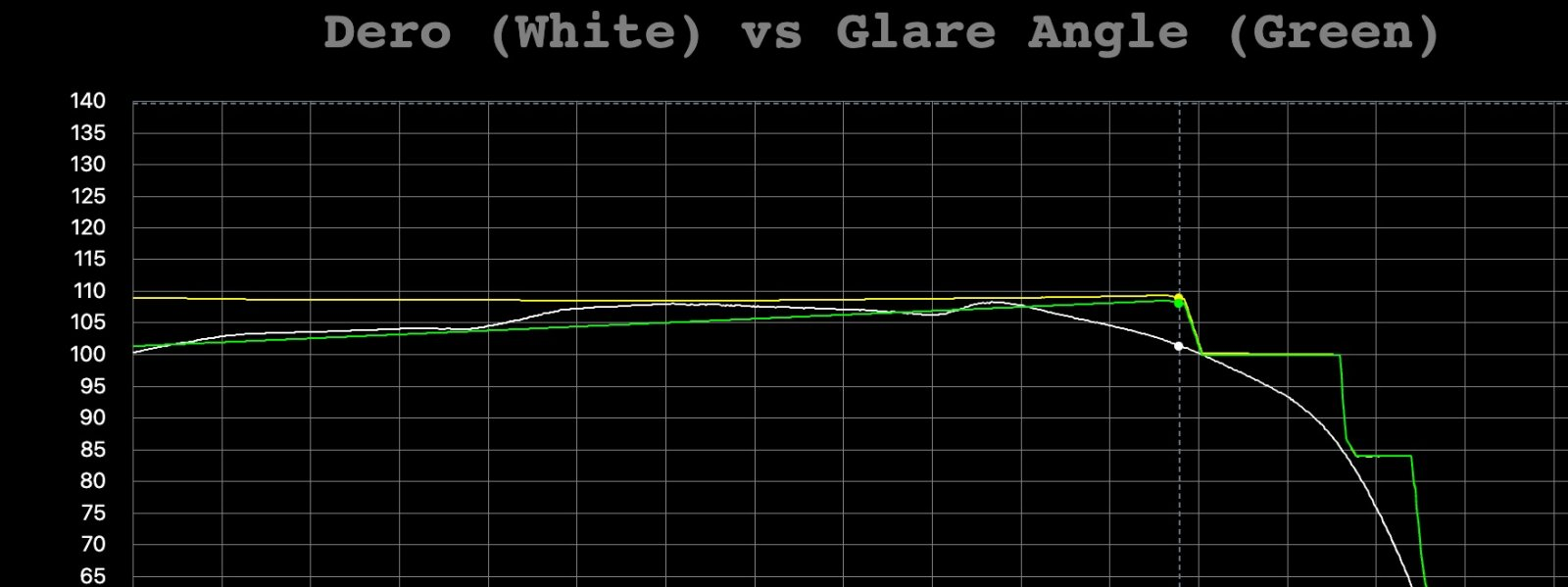The calculated pod roll (yellow) is pretty flat, which means I could have just left it at that value, and we'd get, essentially, the same thing.

What version should we use then, the new one? Also, what is the "Scale jet pitch with roll" option?

What version should we use then, the new one? Also, what is the "Scale jet pitch with roll" option?
The new one is probably closest to reality, although I'm not super happy with it. It's a refinement but bumps up against the low quality of the recorded data - mostly the difficulty in getting an accurate cloud velocity track. But using the simply ramp, or calculating it based on the old movements yields essentially the same result. And really changes nothing after the first 22 seconds (when dero and pod roll are essentially identical.)

Again, it's a refinement that does not change the observables - and in fact, demonstrates an even closer correlation of the model and the rotating glare hypothesis with the data. With or without refinement, the basic results are unchanged.

"Scale jet pitch with roll" has been there for some time. It adjusts the jet pitch to maintain constant lift when rolling (banking). It does not make a huge difference as the roll does not change much.

JavaScript:
``````if (par.scaleJetPitch) {
var roll = jetRollFromFrame(f)
}``````

Shouldn't the error angle graph be reverted to something like the old version ? It's supposed to approximate the difference between where the pod is actually looking without the steering mirrors and where it should be looking in order to track the object. But now it depends on the desired horizon angle as well which you agree probably shouldn't cause additional pod roll ? Previously it still depended on the glare angle which is also incorrect, but the keyframed glare angle graph (with the step added during the first seconds) was probably a good approximation for the stepped behavior of the pod roll as the resulting error graph was really good at making sense of all the bumps and sudden rotations. Maybe it'd be better to try to write an algorithm to predict the stepped pod roll behavior without looking at the glare angle, only the location of the bumps perhaps to resolve some ambiguity, but until we have such a thing, the previous error angle graph was better ?

Last edited:
It's supposed to approximate the difference between where the pod is actually looking without the steering mirrors and where it should be looking in order to track the object.
It's measuring that angle in the sim, i.e the angle between the white dot (the target) and the green dot (the physical pointing direction of the pod). It (the magenta graph at the bottom) is not hugely different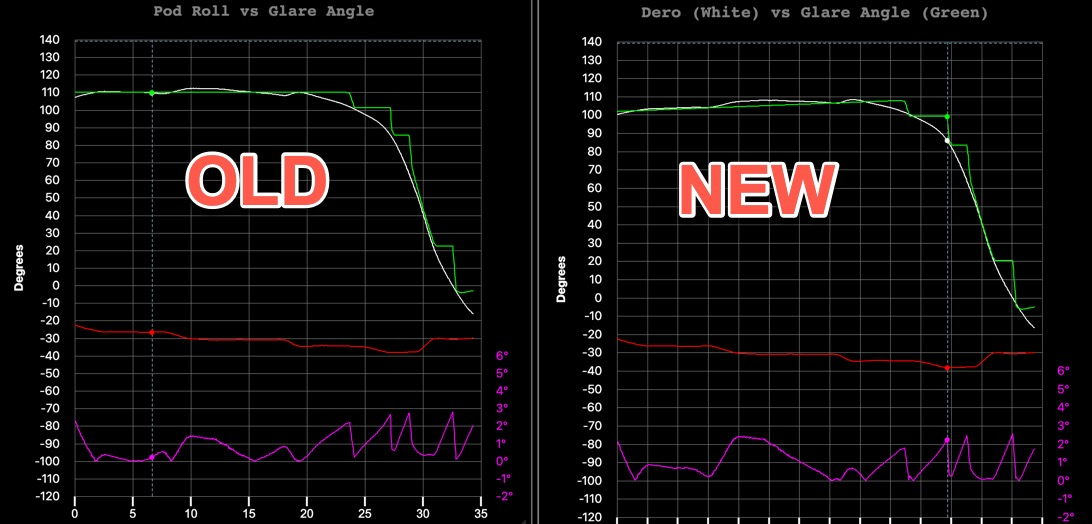There's a couple of factors here. I'm using the glare angle to drive the pod roll in both, but previously it was just glare angle = pod roll, and now it's glare angle = derotation to get the desired horizon, from which we can calculate pod roll. I've also shoehorned in a 6° constant rotation of the glare.

For glare to drive roll (or glare->dero->roll) we need a "glare start angle", as we can't determine the 1:1 relationship, only the delta values (i.e. a change in glare should produce a corresponding change in roll. This value is arbitrary, and you can adjust it in Tweaks/Glare Start Angle. But I have to give it value.
In the old code it was simply the average of the ideal roll for the first 22 seconds (so the green line goes through the middle of the white line)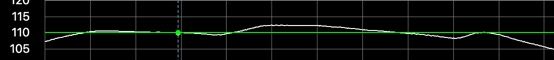In the new code I pick a value that attempts to minimize the largest error over the entire track. Similar end result: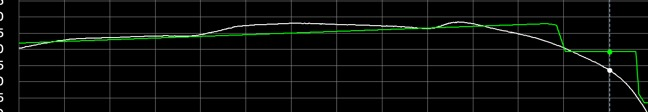However, as you can see above, this now reduces the largest error to 2.5°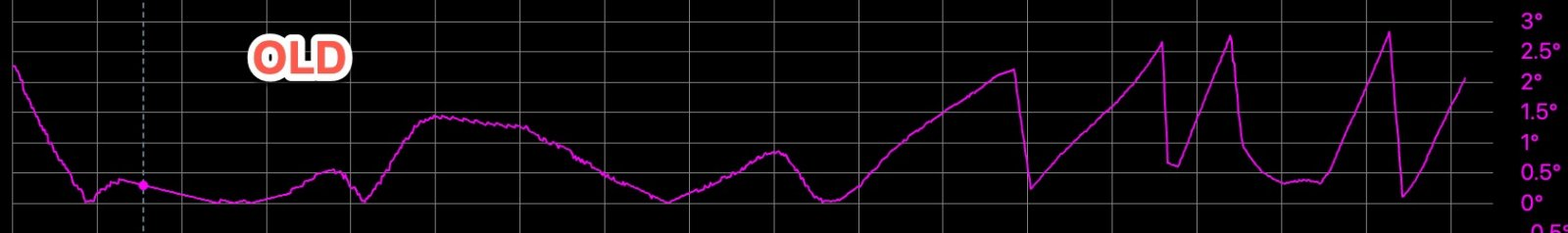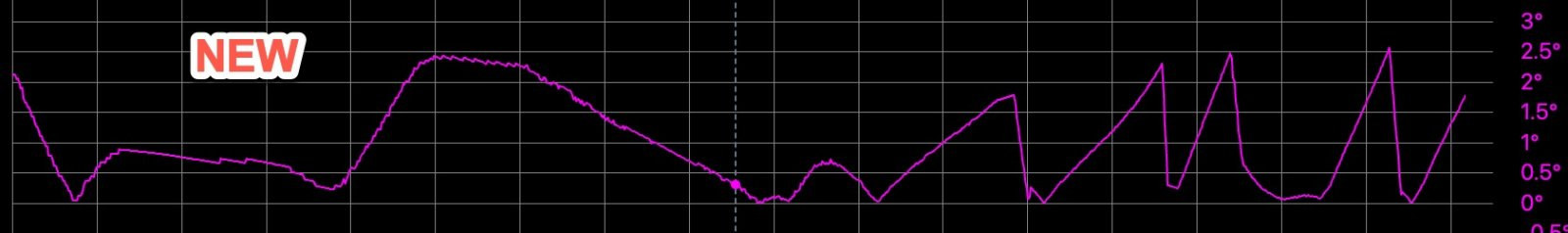However, you could also get pretty much the same result in the old code.

In the new code I pick a value that attempts to minimize the largest error over the entire track.
JavaScript:
``````function calculateGlareStartAngle() {

var n = 650;
var errSum = 0
var glareSum = 0;
for (var f=0;f<n;f++) {
errSum += getGlareAngleFromFrame(f)-podRollFromFrame(f)
glareSum += getGlareAngleFromFrame(f)
}
var avg = errSum/n // the average difference is the adjustment needed to make it zero
var glareAvg = glareSum/n

// this is a bit ad-hoc and does not really work
par.glareStartAngle -= avg -  (getGlareAngleFromFrame(0) - glareAvg)

// use that as a start, and now refine it to minimize the maximum error
// this is a somewhat expensive (slow) operation

var bestError = 100000
var bestStartAngle = par.glareStartAngle

var start = par.glareStartAngle-5
var end = par.glareStartAngle+5
for (var angle = start; angle < end; angle+=0.2) {
par.glareStartAngle = angle; // set it temporarily to see if it's any good
var biggestError = 0
for (var frame = 0; frame < Sit.frames; frame += 5) {
var podRoll = podRollFromFrame(frame); // this is JUST the roll not from
var pitch,globalRoll;
[pitch, globalRoll] = pitchAndGlobalRollFromFrame(frame)

var glarePos = PRJ2XYZ(pitch, getPodRollFromGlareAngleFrame(frame) + jetRollFromFrame(frame), jetPitchFromFrame(frame), vizRadius)

var v = PRJ2XYZ(pitch, globalRoll, jetPitchFromFrame(frame), vizRadius)
var errorAngle = degrees(v.angleTo(glarePos))
if (errorAngle > biggestError)
biggestError = errorAngle
}
//console.log("Angle = "+angle+": biggestError = "+biggestError)
if (biggestError < bestError) {
bestError = biggestError;
bestStartAngle = angle
// console.log("bestStartAngle = "+bestStartAngle)

}
}
par.glareStartAngle = bestStartAngle

}``````

It's not perfect, as it's an expensive operation so I do it at low resolution.

If one wants to explain all the bumps by pod roll, this error angle graph does not explain the bump at 1s (error peak occurs before 1s), and the model predicts a bump at 10s that does not happen.

I'm intrigued by this step-rotating pod. First because I don't see how it put less constraint on the pod, every ~3deg error the motors need to kick in, creating bumps/steps in the image. And also because if it was something that happens all the time, and not only at singularity (between -3 and 3°Az), this would be a very common feature of ATFLIR, that we would see in at least some examples. I've only seen smooth rotations so far (like in DCS). Experts/pilots would be very familiar with it too.

Except it was a specific algorithm tweak for this generation of ATFLIR. @Mick West , did you ever contact John Ehrhart, the ATFLIR expert that talked to Corbell?

Except it was a specific algorithm tweak for this generation of ATFLIR. @Mick West , did you ever contact John Ehrhart, the ATFLIR expert that talked to Corbell?
Do you have his email address?

No, but he's on LinkedIn, maybe he could be contacted there.

this now reduces the largest error to 2.5°
As mentioned previously, now you have one more point, at 10 seconds, where the error seems to reach 2.5 but does not result in any noticeable bump or sudden glare rotation. Maybe you could say in reality it doesn't actually reach 2.5, just gets really close to it, but the previous version didn't have this issue at all. Also, the error peak at 24 seconds moves from 2.2 degrees to about 1.7 degrees, so that one already had a bit of a keyframing bug on it but now it moved even further from 2.5. We can't know for sure that they have to line up at the same value, but it makes a lot more sense if there's a common error threshold for the pod to start rolling. That some of the peaks were slightly higher than 2.5 previously seems like a lesser problem that could be due to other factors as well.
you could also get pretty much the same result in the old code.
This is what I get if I use the new default glare start angle (59.157..) in my version of the old sim. In the second half of the video it's similar but something has changed in the first half.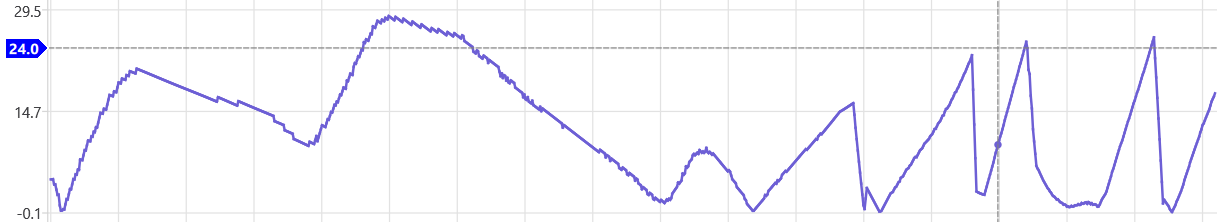Maybe the new calculated pod roll graph is pretty flat but not flat enough ? I can't fiddle with the gimbal sim for too long as it overheats my laptop so I still need to implement the new changes in my version to be able to experiment more freely. The new getPodHorizonFromJetAndPod function is a bit of a headache to implement for me as the 3D model is in the GUI layer, not the backend layer. Have you checked if doing it that way is noticeably different than just rotating some vectors to get the pod horizon ?

I can't fiddle with the gimbal sim for too long as it overheats my laptop
Just FYI Mick, I have the same problem with the Gimbal sim and Sitrec. It's great there is so much stuff but it's very laggy on my laptop.

I have the same problem with the Gimbal sim and Sitrec.
Sitrec should be fixed, performance-wise.

Gimbal sim next.

is [getPodHorizonFromJetAndPod] noticeably different than just rotating some vectors to get the pod horizon ?
Turns out the answer to that was no, it's just equivalent to rotating some vectors.

So now I've added all of these changes to my version and I get the same error angle graph: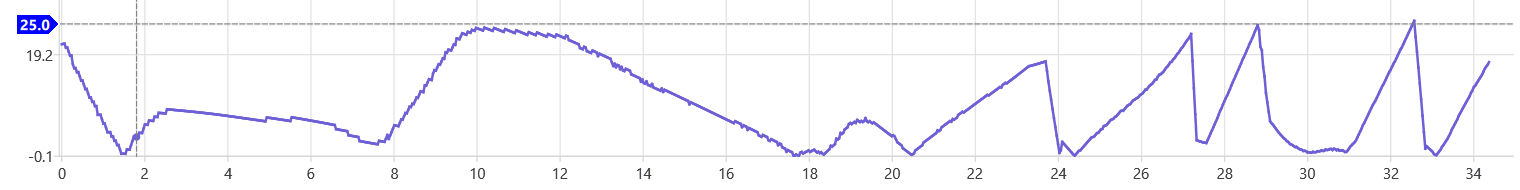It's probable that there's an analytical solution to finding roll from dero.
As an exercise to help me understand the math here I wrote an analytical solution for it. It's always within 0.05 degrees of the horizon angle that the binary search finds.
C++:
``````// a x^2 + b x + c = 0
std::pair<double, double> solve_ax2_bx_c(double a, double b, double c) {
double delta = b * b - 4 * a * c;
double x1 = (-b + sqrt(delta)) / (2 * a);
double x2 = (-b - sqrt(delta)) / (2 * a);
return { x1, x2 };
}

//a cos x + b sin x + c = 0
std::pair<double, double> solve_acosx_bsinx_c(double a, double b, double c) {
// rewrite as x = cos x
// a x + b sqrt(1-x^2) + c = 0
// a x + c = -b sqrt(1 - x^2)
// a^2 x^2 + 2 a c x + c^2 = b^2 (1 - x^2)
// x^2 (a^2 + b^2) + 2ac x + (c^2 - b^2) = 0
auto [cos_x1, cos_x2] = solve_ax2_bx_c((a * a + b * b), 2 * a * c, (c * c - b * b));
return { acos(cos_x1), acos(cos_x2) };
}

// based on extractRollFromMatrix but using a formula for the signed angle to avoid branching
double pod_horizon_from_bases(vec3d podUp, vec3d podForward, vec3d vy = { 0,1,0 }) {
auto podFwdY = podForward.cross(vy).cross(podForward);
return degrees(atan2(podUp.cross(podFwdY).dot(podForward), podUp.dot(podFwdY)));
}

double pod_roll_from_horizon_analytic(double jetRoll, double jetPitch, double podPitch, double horizonTarget) {
vec3d vx = { 1, 0, 0 }, vy = { 0, 1, 0 }, vz = { 0, 0, 1 };

// Given jetRight,jetForward after applying jetRoll,jetPitch we have:
// podRight = jetRight.rotate(jetForward, -podRoll)
// podForward = jetForward.rotate(podRight, -podPitch)
// podUp = podForward x podRight
// podFwdY = podForward x vy x podForward // based on extractRollFromMatrix
// horizon_angle = podUp.angleTo(podFwdY)
//   = atan2((podUp x podFwdY) . podForward, podUp . podFwdY)
// (podUp x podFwdY) . podForward = tan(horizon_angle) (podUp . podFwdY)  -- (1)

// It's easier to solve these equations in the frame of reference of the jet.
// jetRight becomes vx, jetForward becomes vz, and so it can be shown that:
// podRight = { cos(podRoll), -sin(podRoll), 0 }
// podUp = { cos(podPitch) sin(podRoll), cos(podPitch) cos(podRoll), -sin(podPitch) }
// and the vy used above becomes:
vec3d vy_jet = vy.rotate(vx, radians(-jetPitch)); // reversed the order and sign of these rotations

// Using (a x b) x c = b (a.c) - a (b.c):  -- https://en.wikipedia.org/wiki/Triple_product#Vector_triple_product
// podFwdY = vy - podForward (vy . podForward)
//
// podUp . podFwdY = (vy.podUp) - (podForward.podUp)(vy.PodForward) = vy.podUp -- (2)
//
// podUp x podFwdY = (podForward x podRight) x podFwdY
// = podRight (podForward . podFwdY) - podForward (podRight . podFwdY)
// = -podForward (podRight . podFwdY)  // since podForward.podFwdY = (vy.podForward) - (podForward.podForward)(vy.podForward) = 0
// = -podForward (vy . podRight) // since podRight.podFwdY = (vy.podRight) - (podForward.podRight)(vy.podForward) = (vy.podRight)
// so (podUp x podFwdY) . podForward = -vy . podRight -- (3)

// (1),(2),(3) => -vy . podRight = tan(angle) vy . podUp   // now we can substitute podRight,podUp from above:
// (v . (cos x, -sin x, 0)) = a * (v . (cos p sin x, cos p cos x, -sin p)) // rename v = vy_jet, a = -tan(angle), p = podPitch, x = podRoll
// vx cos x - vy sin x = a * (vx cos p sin x + vy cos p cos x - vz sin p ) // rename vx = v.x, vy = v.y, vz = v.z
// cos x (vx - a vy cos p) - sin x (vy + a vx cos p) + a vz sin p = 0
// and now this is an equation in x that we can solve:

auto [podRoll_1, podRoll_2] = solve_acosx_bsinx_c(
vy_jet.x + tan_horizon * vy_jet.y * cos(podPitch_rad),
-(vy_jet.y - tan_horizon * vy_jet.x * cos(podPitch_rad)),
);

// acos returns the absolute value of the angle, so try all possible signs
double podRoll_results[] = { podRoll_1, -podRoll_1, podRoll_2, -podRoll_2 };
double podRoll = podRoll_1;
double err_min = std::numeric_limits<double>::max();
for (int i = 0; i < 4; i++) {
double x = podRoll_results[i];
double horizon = pod_horizon_from_bases(podUp, podForward, vy_jet);
double err = abs(horizon - horizonTarget);
if (err < err_min) {
podRoll = x;
err_min = err;
}
}
return degrees(podRoll);
}``````

Last edited:
Nice work, just a quick question. Has anyone analysed the other gimbal video if the “ufo” flying low over the ocean? this is when they get a lock on it.